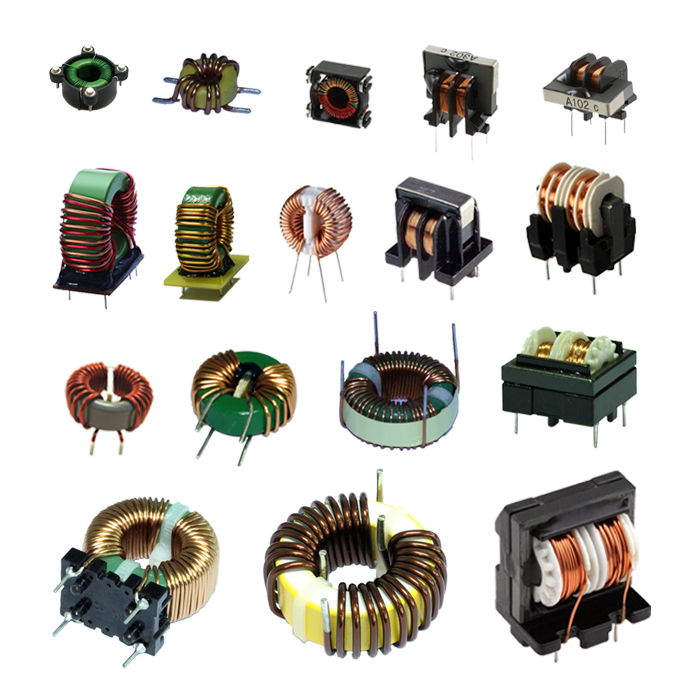# Four main role of inductors

##### author: MagTop
01/06/2022The role of inductors 1.
Inductance coil blocking effect: the self-inductive electromotive force in the inductive coil coil is always resistant to the current change in the coil. The inductive coil has a blocking effect on the alternating current, and the size of the blocking effect is called the inductive reactance XL, and the unit is ohm. Its relationship with inductance L and AC frequency f is XL=2πfL. Inductors can be mainly divided into high-frequency choke coils and low-frequency choke coils.
The role of the inductor 2.
Tuning and frequency selection: the inductance coil and the capacitor are connected in parallel to form an LC tuning circuit. That is, the natural oscillation frequency f0 of the circuit is equal to the frequency f of the non-AC signal, then the inductive reactance and capacitive reactance of the loop are also equal, so the electromagnetic energy oscillates back and forth in the inductor and capacitor, which is the resonance phenomenon of the LC loop. During resonance, the inductive reactance and capacitive reactance of the circuit are equal and opposite, the inductive reactance of the total loop current is the smallest, and the current amount is the largest (referring to the AC signal with f="f0"). An AC signal of frequency f is selected.
The role of the inductor 3.
Inductors also have the functions of filtering signals, filtering noise, stabilizing current and suppressing electromagnetic wave interference.
The role of the inductor 4.
In electronic equipment, it is often seen that there is a magnetic ring as shown in Figure 2. What are the functions of these small things? This magnetic ring and the connecting cable form an inductor (the wire in the cable is wound around the magnetic ring several times with an inductive coil), It is a commonly used anti-interference component in electronic circuits. It has a good shielding effect on high-frequency noise, so it is called an absorption magnetic ring. It is usually made of ferrite material, also known as ferrite magnetic ring (referred to as magnetic ring). . In Figure 2, the above is a one-piece magnetic ring, which is a magnetic ring with a mounting clip. The magnetic ring has different impedance characteristics at different frequencies. The impedance is very small at low frequencies, and the impedance of the magnetic ring increases sharply when the signal frequency increases.